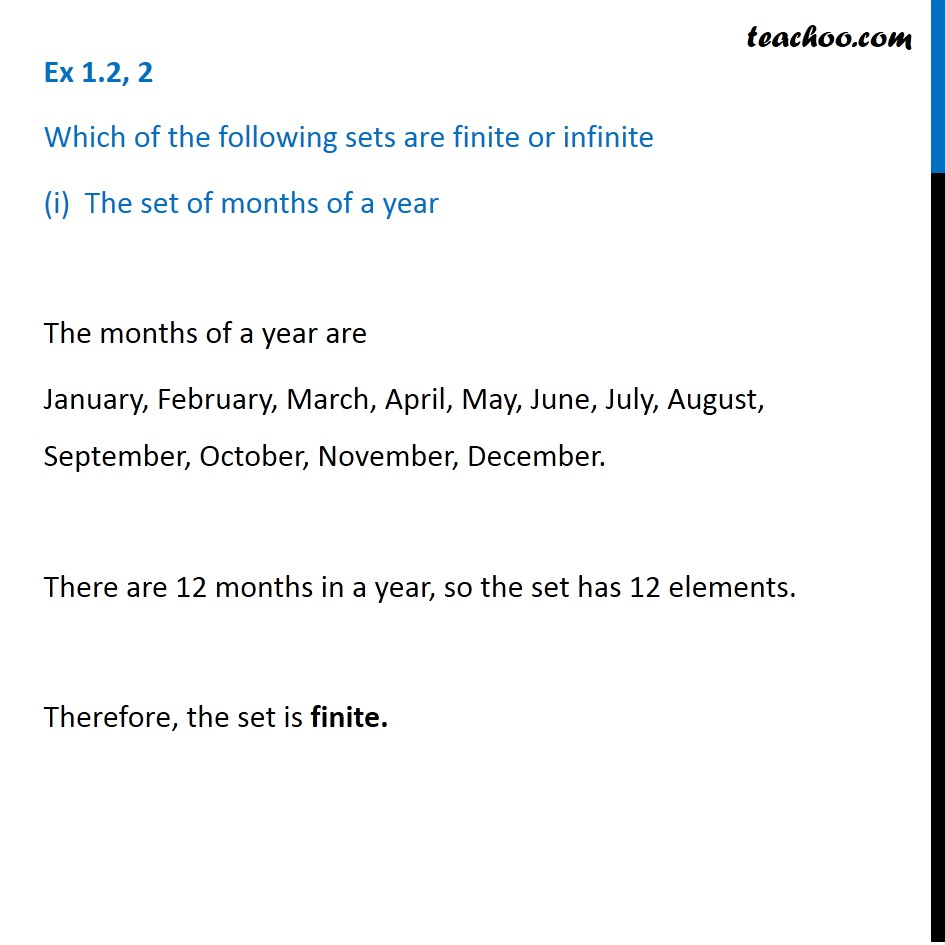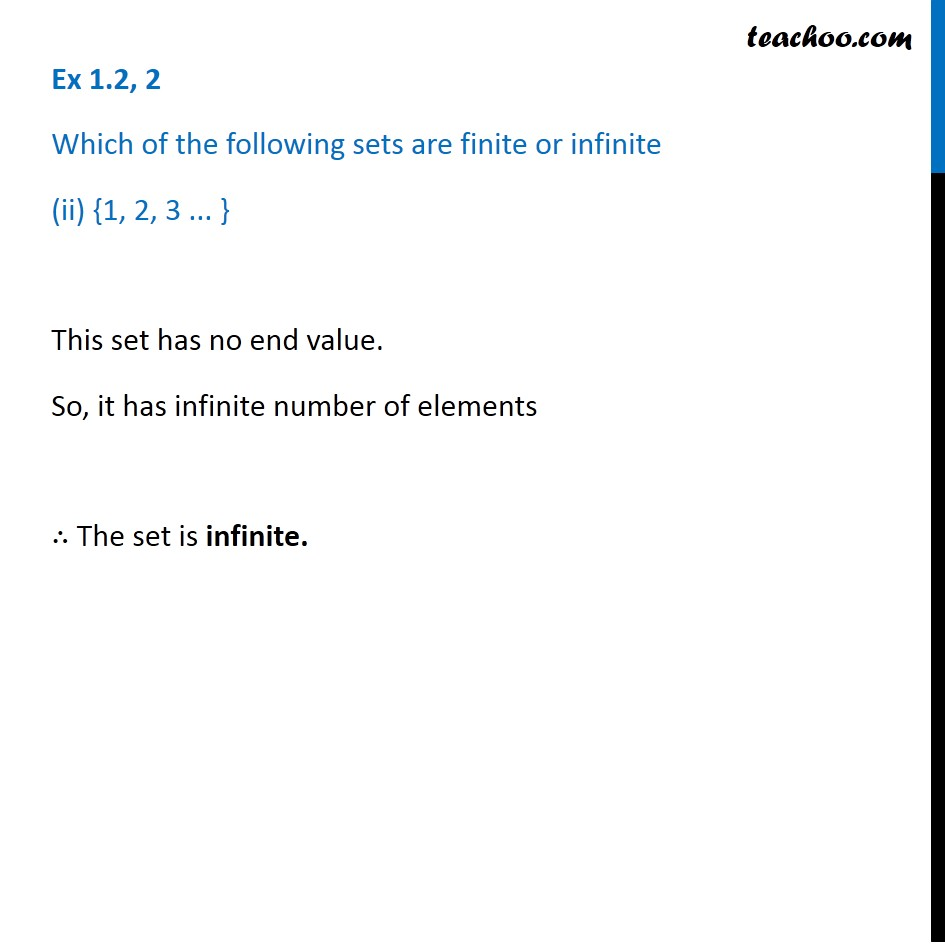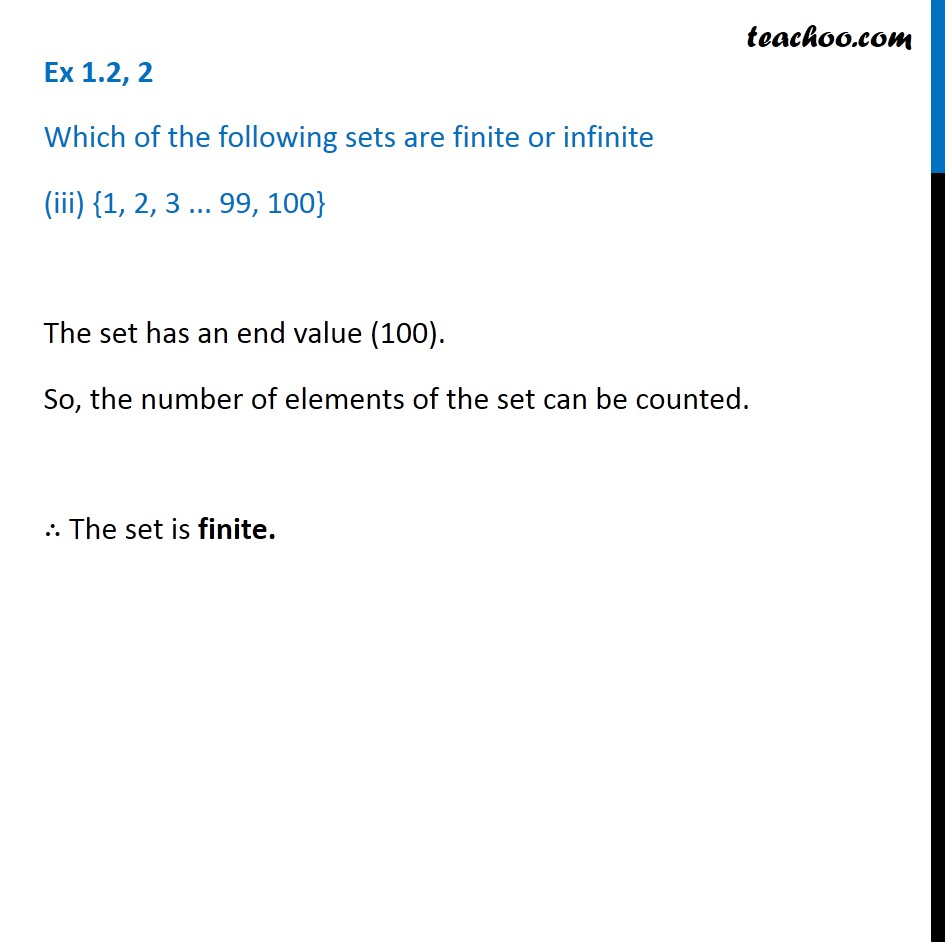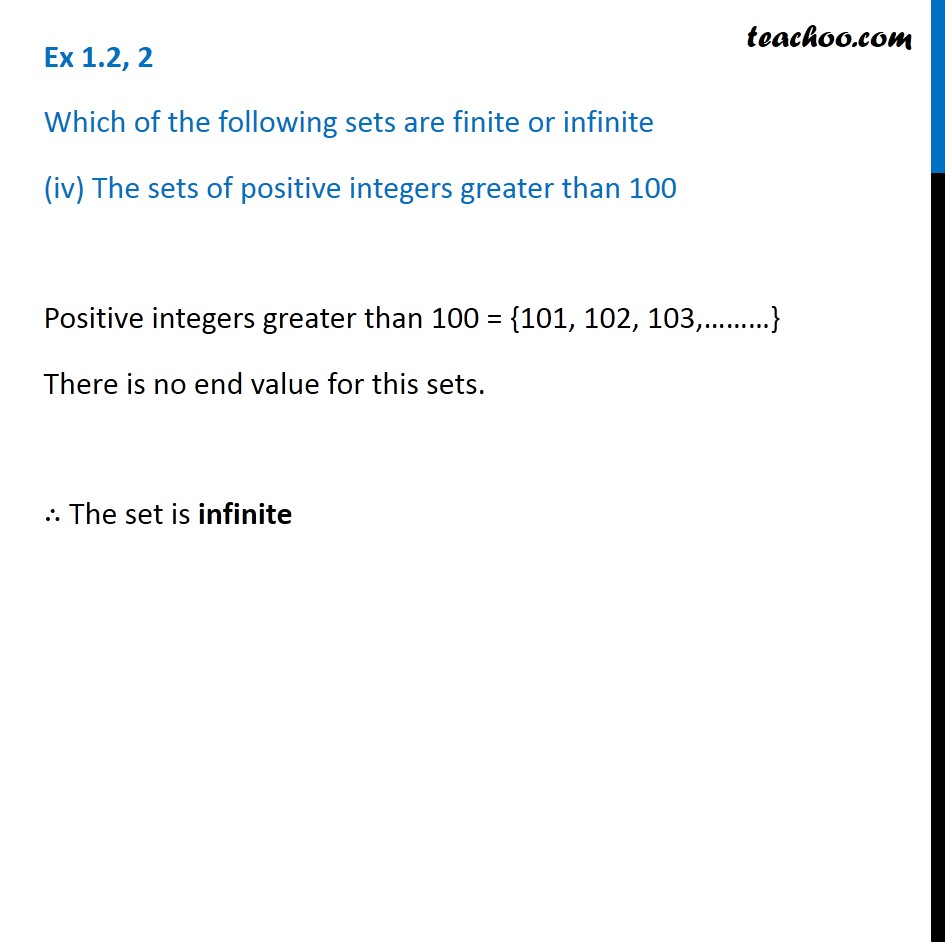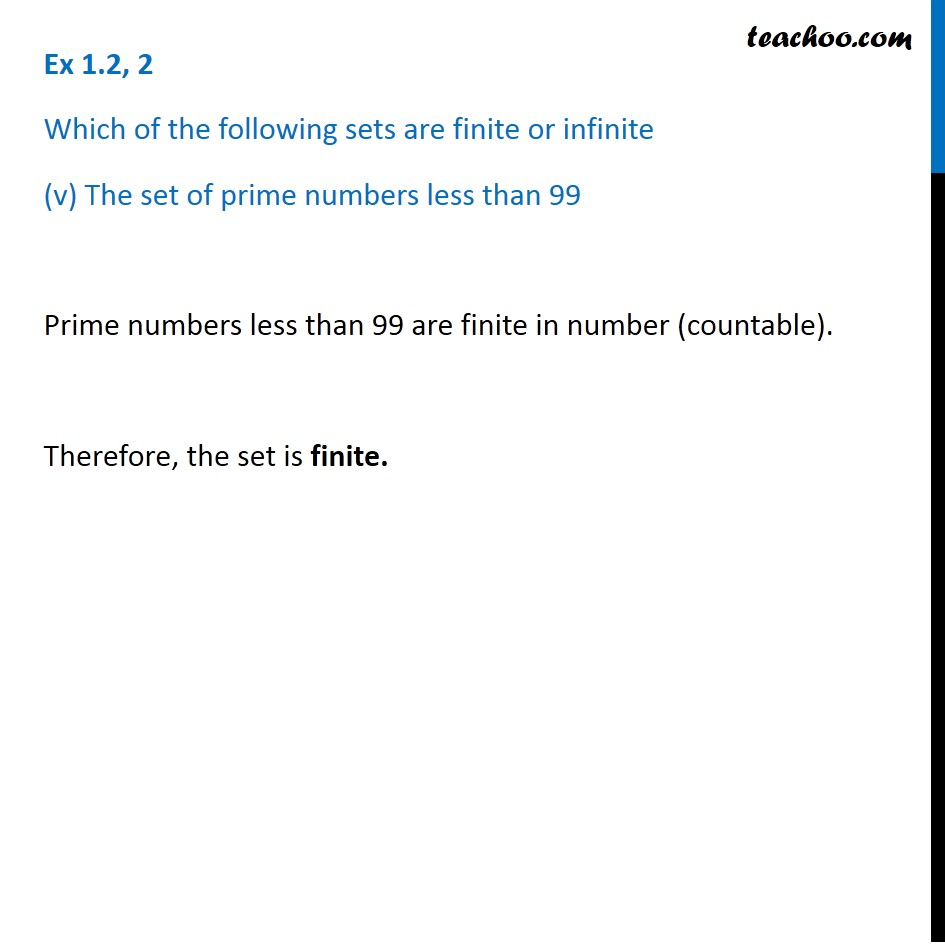1. Chapter 1 Class 11 Sets
2. Serial order wise
3. Ex 1.2

Transcript

Ex 1.2, 2 Which of the following sets are finite or infinite (i) The set of months of a year The months of a year are January, February, March, April, May, June, July, August, September, October, November, December. There are 12 months in a year, so the set has 12 elements. Therefore, the set is finite. Ex 1.2, 2 Which of the following sets are finite or infinite (ii) {1, 2, 3 ... } This set has no end value. So, it has infinite number of elements ∴ The set is infinite. Ex 1.2, 2 Which of the following sets are finite or infinite (iii) {1, 2, 3 ... 99, 100} The set has an end value (100). So, the number of elements of the set can be counted. ∴ The set is finite. Ex 1.2, 2 Which of the following sets are finite or infinite (iv) The sets of positive integers greater than 100 Positive integers greater than 100 = {101, 102, 103,………} There is no end value for this sets. ∴ The set is infinite Ex 1.2, 2 Which of the following sets are finite or infinite (v) The set of prime numbers less than 99 Prime numbers less than 99 are finite in number (countable). Therefore, the set is finite.

Ex 1.2

Chapter 1 Class 11 Sets
Serial order wise